# Hinges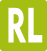RIGHT & LEFT SIDE OPENING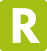RIGHT SIDE OPENING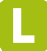LEFT SIDE OPENING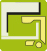EXTERNAL HINGE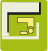CONCEALED HINGE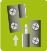LIFT-OFF HINGE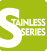STAINLESS SERIES
IMAGE
PRODUCTSDETAILS

X
X
X
X

X
X
X
X

X
X
X
X

X
X
X
X

X
X
X
X

X
X
X
X
X

X
X
X
X

X
X
X
X
X

X
X
X
X

X
X
X
X
X

X
X
X
X
X

X
X
X
X

X
X
X
X

X
X
X
X
X

X
X
X
X

X
X
X

X
X
X
X

X
X
X
X
X

X
X
X
X
X

X
X
X
X
X

X
X
X
X
X

X
X
X
X
X

X
X
X
X
X
X
X

X
X
X
X
X

X
X
X
X
X

X
X
X
X
X

X
X
X

X
X
X
X

X
X
X
X

X
X
X
X

X
X
X
X

X
X
X
X

X
X
X
X

X
X
X
X
X

X
X
X
X

X
X
X
X

X
X
X
X

X
X
X
X

X
X
X
X

X
X
X
X

X
X
X
X

X
X
X
X
X

X
X
X
X
X

X
X
X
X
X

X
X
X
X
X

X
X
X
X
X

X
X
X
X
X

X
X
X
X
X

X
X
X
X
X

X
X
X
X
X

X
X
X
X
X

X
X
X
X
X

X
X
X
X

X
X
X

X
X
X
X
X

X
X
X
X
X

X
X
X
X

X
X
X
X
X

X
X
X

X
X
X

X
X
X
X

X
X
X

X
X
X

X
X
X

X
X
X

X
X
X
X
X
X
X

X
X
X

X
X
X

X
X
X
X
X

X
X
X
X
71. ## Concealed Hinge - 2414

X
X
X
X
X
X
X

Contact Metrol Springs Limited on 01604 499332.
Alternatively, click here to contact via email or via our fax on 01604 493390.